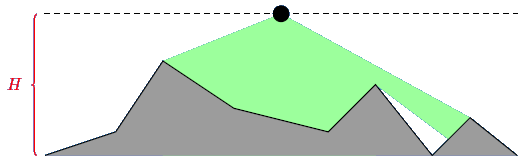시간 제한 메모리 제한 제출 정답 맞은 사람 정답 비율
1 초 512 MB 3 3 3 100.000%

## 문제

The Smurfs have built the Great Smurfy Wall.  The wall consists of a sequence of points connected by straight wall segments. Smurfs have also built towers on some of those points.  Now Gargamel needs to observe all those towers, but he can't do this himself because wall segments can obscure his vision (although it is possible to see all towers along the wall).  He wants to place some cameras along a highway that passes nearby the wall.  However the cameras are very expensive so Gargamel asked you to tell him the minimal number of cameras he needs.## 입력

First line of input contains integers $n$ and $H$ ($1 \leq n \leq 10^5$, $1 \leq H \leq 10^6$). $n$ is the number of points of the wall, and $H$ specifies that highways is the line with $y = H$. The next $n$ lines describe the Great Smurfy Wall.  Each of those lines contains three integers $x_i, y_i, z_i$ ($0 \leq x_i \leq 10^6$, $0 \leq y_i < H$, $z_i \in \{0,1\}$), $(x_i, y_i)$ are the coordinates of a point, and $z_i = 1$ iff there's a tower at that point.  $y_1$ and $y_n$ are always $0$, and the points are given in the order of strictly increasing $x_i$.

## 출력

Output the minimal number of cameras Gargamel needs to use.

## 예제 입력 1

9 30
0 0 1
15 5 1
25 20 1
40 10 1
60 5 1
70 15 1
82 0 1
90 8 1
100 0 1


## 예제 출력 1

2